Courses

# Solid Liquid Equilibria Civil Engineering (CE) Notes | EduRev

## Civil Engineering (CE) : Solid Liquid Equilibria Civil Engineering (CE) Notes | EduRev

The document Solid Liquid Equilibria Civil Engineering (CE) Notes | EduRev is a part of the Civil Engineering (CE) Course Thermodynamics.
All you need of Civil Engineering (CE) at this link: Civil Engineering (CE)

Solid-Liquid Equilibria

The prediction of solubility of a solid in a liquid is important for design of separation processes that utilize either preferential dissolution or crystallization as a route to purification of a species. The presence of a dissolved solid in a liquid also changes the freezing (as well as boiling) point of the latter, and its estimation is important for design of heat exchange equipments such as crystallizers (and evaporators). A wide variety of phase behaviour has been observed in systems which comprise mixtures of solids, mixture of a solid and a liquid and mixtures comprised of a liquid and more than a single solid. This section is devoted to consideration of thermodynamic relations that allow computation of the solubility of solids in liquids as a function of temperature. Such relations may also be extended to estimate changes in freezing or boiling point of a liquid in presence of dissolved solids.

The phase equilibria relations developed here are limited to the simplest case of systems comprising a solid (1) and a liquid (2) (solvent). As with all other phase equilibria, one starts with the basis of equality of the species fugacity in the solid (s) and liquid (l) phases respectively.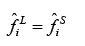..(9.37)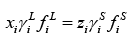..(9.38)

Where, xi and zi are, respectively, the mole fractions of species ‘i’ in the liquid and solid solutions. Equivalently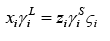..(9.39)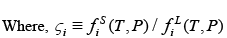..(9.40)

The right side of this equation, defines ψi as the ratio of fugacities at the temperature T and pressure P of the system. Note that for each pure species at its (say, normal) melting point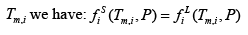..(9.41)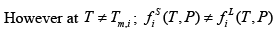Thus, in general one needs to derive a suitable expression for ς i defined by eqn. 9.40. This may be done by through estimation of the enthalpy and entropy of fusion at the system temperature T, with values of the enthalpy and entropy at the melting point at the system pressure as datum. However, since the properties of solids are not dependent on pressure in a significant way, for convenience of computation one can assume the reference point as the normal melting point Tm,i . Accordingly, we may write for either the solid or the solvent species: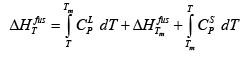..(9.42)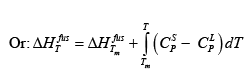..(9.43)

In the same manner one may write: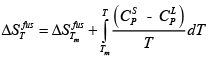..(9.44)

At temperature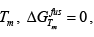thus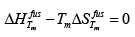..(9.45)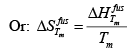..(9.46)

Thus, using eqn. 9.46 in 9.44 we have: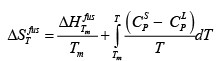..(9.47)

Further, at temperature T, we have: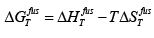..(9.48)

Therefore, substituting eqns. 9.43 and 9.47 in 9.48 and re-arranging we have: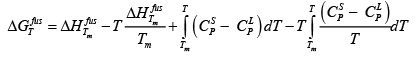..(9.49)

If we further make the simplifying assumption that ( CPS −CPL ) is constant over the temperature range Tm toT one obtains: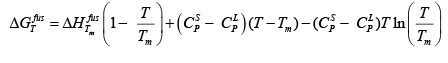..(9.50)

Now,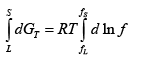or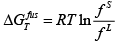..(9.51)

Thus, comparing eqn. 9.50 and 9.51: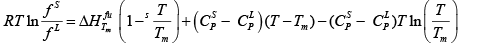..(9.52)

Therefore using eqn. 9.52 in eqn. 9.40, we have:..(9.53)

Upon suitable rearrangement: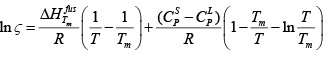..(9.54)

Now by eqn. 9.39: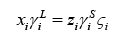..(9.39)

With ςi given by the general expression as in eqn. 9.54, a suitable activity coefficient model may be chosen to represent the activity coefficients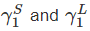in the solid and liquid phases, respectively and then eqn. 9.39 may be solved to yield the phase compositions. We illustrate the typical methodology of such calculations for a binary system. Let us assume a solid (1) is to be solubilized in a liquid (2). The starting point is eqn. 9.39. However, in applying it we make the simplifying assumption that the liquid does not “dissolve” in the solid phase, and so the latter phase is a pure component solid. This is a reasonable approximation for many system of practical interest in the chemical industry. The liquid phase, however, will be a binary with the solid transforming to a “liquid” phase and then mixing with the liquid solvent. Thus, one may rewrite the phase equilibrium as: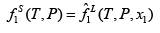..(9.55)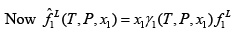..(9.56)

Thus from the last two equations, the equilibrium solubility of the solid ( x1) in the liquid is given by: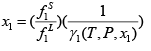..(9.57)

It follows that if the temperature at which the solubility is desired is the melting point Tm of the solid, then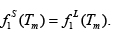..(9.58)

Therefore, we have: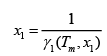..(9.59)
However, for any temperature T (other than T) eqn. 9.58 needs to be employed. Thus, we have:

In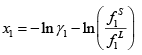..(9.60)

Or using eqns. 9.40 and 9.52 (or 9.53) we have: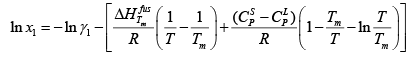..(9.61)

One may solve eqn. 9.61 (iteratively) for the solubility x1 , by assuming a suitable activity coefficient model for the liquid phase (for ex: van Laar, Regular Solution, NRTL, etc). In the special case where the temperature of interest T is close to the melting point Tm so that one can make the approximation TTm ≈ 1 eqn. 9.61 reduces to: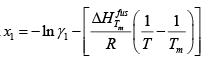..(9.62)

For the purpose of further illustration of the results that typically obtain from application of the foregoing phase equilibria relations, we consider two limiting or special cases for a binary system assuming that eqn. 9.62 applies. It may be noted, however, that this assumption is not essential to the considerations below. Using eqn. 9.54 we write: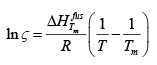..(9.63)

Or, for any of the species: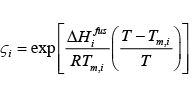..(9.64)

The limiting cases are:

1. Both phases form ideal solutions, i.e.,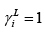and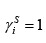for all temperature and compositions.

2. The liquid phase behaves as an ideal solution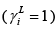, and all species in the solid state are completely immiscible, i.e.,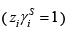Case 1

Writing eqn. 9.39 for both species, we have:

x1 =z1 ς1
x1 =z1 ς1                             ..(9.65)

Further:
x1 +x2= 1                         ..(9.67)

z1 +z2 = 1                          ..(9.68)

Using eqns. 9.57 – 9.60, one obtains: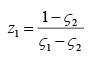..(9.69)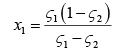..(9.70)

If one uses the simplified form forς , then by eqn. 9.64: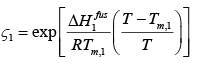..(9.71)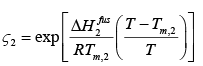.(9.72)

On putting x1 =z1 = 1 it follows that ς1 = 1; thus, by eqn. 9.71, T =Tm,1. In the same manner if we put x2 =z2 = 1 then ς= 1; and T =Tm,2 . On choosing a series of temperature T between Tm,1 and Tm,2 and for each case solving for xi andzi one obtains a T − x−z plot that is shown schematically in fig. 9.4. The upper curve in fig. 9.4 is the freezing curve and the lower curve is the melting curve. Any T −x point within the lens-shaped region between the two curves corresponds to a two phase situation, while points outside the region depict a single phase. If a point is above the upper curve the state of the system is a homogeneous binary liquid mixture, whereas if the point lies beneath the melting curve, the state of the system is binary solid mixture. Any straight line parallel to the composition axis depicts a tie line (for example XY), the compositions at the two ends signifying the liquid and solid phase compositions. This behaviour is analogous to that of Raoult’s law T − x− y plot shown in fig.7.7.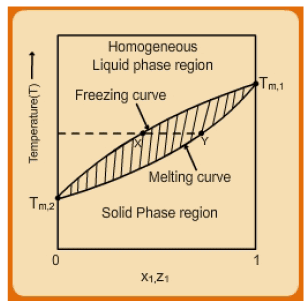Figure 9.4 Case I, ideal liquid and solid solutions

An example of a system exhibiting a phase diagram of this type is a binary mixture of nitrogen/carbon monoxide at low temperatures. However, as may be evident, this phase behaviour is necessarily an idealized one. Its utility lies in that it can provide a reference system for interpreting the behviour of more complex, real systems.

Case 2

For this case the liquid phase forms ideal solution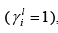, and there is complete immiscibility for all species in the solid state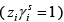. Thus the governing phase quilibria relations are:

x2 = ς2                              ..(9.73)

x2 = ς2                              ..(9.74)

Since, ς1 andς2 are functions of temperature alone (by eqns.9.71 and 9.72), it follows that x1 and x2 likewise are functions of temperature only. Thus eqns. 9.73 and 9.74 may be solved independently upon which one obtains two distinct plots on the T −x1 diagram as shown in fig. 9.5. However, if eqns. 9.64 and 9.65 apply simultaneously, then we have:ς1 + ς2= 1 and hence x1 +x2= 1. Thus, it follows that under this condition the following relation holds: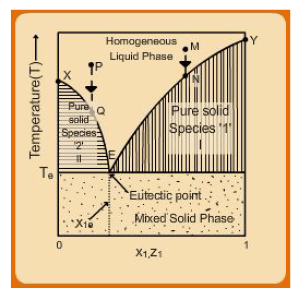Fig. 9.5 T-x-z plot for Case II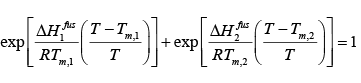(9.75)

On solving the above equation one obtains the Eutectic temperature Te shown in fig. 9.5. This particular phase diagram is to be interpreted as follows. When eqn. 9.73 applies one has: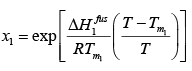..(9.76)

This equation is valid over the temperature range T = Tm1 , and the corresponding composition range x1e <x< 1, where x1 =x1e the eutectic composition. Thus in this region (I) of the phase diagram a liquid solution can be in equilibrium with only pure species 1 as a solid phase. This is represented by region I on fig. 9.5 where liquid solutions with compositions x1 given by line YE are in equilibrium with pure solid 1. Consider the system to be initially at a state defined by point ‘M’. If one cools the system it eventually reaches the point ‘N’ on the T −xplot, at which condition only the component ‘1’ just begins to freeze. This happens till one reaches the eutectic temperature Te at which point component ‘2’ begins to co-precipitate. In the same manner region II obtains by solving eqn. 9.74, i.e.,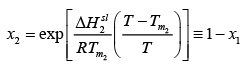This equation is valid only from T = Tm 2 , where, x1 = 0 , to T = Te , where x1 =x1e , the eutectic composition. Equation 9.77 therefore applies where a liquid solution is in equilibrium with pure species 2 as a solid phase. This is represented by region II on fig. 9.5 where liquid solutions with compositions x1 given by line XE are in equilibrium with pure solid 2. Consider an initial system state defined by point ‘P’ which is a binary solution. On cooling, the system eventually reaches the point ‘Q’ where only pure solid ‘2’ begins to crystallize. On continued cooling, the eutectic temperature is reached, whereupon component ‘1’ also precipitates simultaneously.

Finally, if one commences cooling from the point R with a solution composition given by x1 =x1e , no precipitation of any solid occurs till the temperature Te (or point ‘E’) is reached; at which state, as mentioned above, eqn. 9.75 holds. This is a state where two solids are in equilibrium with the solution. Further cooling below Te results in co-precipitation of both species, and the system enters a state where two immiscible solids are present.

Offer running on EduRev: Apply code STAYHOME200 to get INR 200 off on our premium plan EduRev Infinity!

## Thermodynamics

56 videos|92 docs|33 tests

,

,

,

,

,

,

,

,

,

,

,

,

,

,

,

,

,

,

,

,

,

;